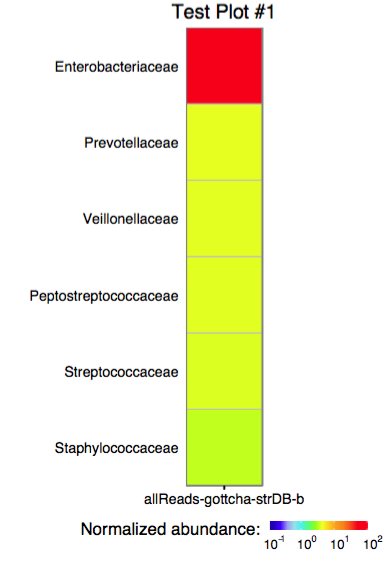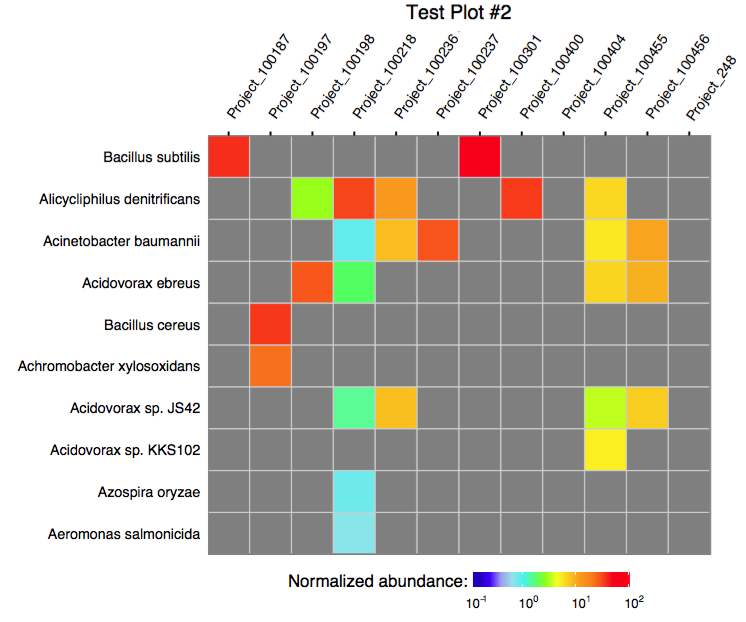# MetaComp

Metagenome taxonomy assignment comparison toolkit. The toolkit is being developed for EDGE platform and reflects its backend specificity. The routines, however, can be used as a stand-alone library for multi-project comparative visualization of taxonomy assignments obtained for metagenomic samples processed with GOTTCHA/GOTTCHA2, BWA, KRAKEN, METAPHLAN, DIAMOND, or PANGIA. The heatmaps can be also visualized with this D3.js-based code which allows to see the exact abundance values in each cell.

#### 0.0 Installation from CRAN

``install.packages("MetaComp")``

to use the library, simply load it into R environment:

``library(MetaComp)``

#### 0.1 Installation from latest sources

``````install.packages("devtools")
library(devtools)
install_github(repo = 'seninp-bioinfo/MetaComp')``````

#### 1.0 Reading a single taxonomic assignment files

``````the_gottcha2_assignment <- load_edge_assignment(data_file_g2, type = 'gottcha2')
the_kraken_assignment <- load_edge_assignment(data_file_k, type = 'kraken')
the_pangia_assignment <- load_edge_assignment(data_file_p, type = 'pangia')``````

#### 1.1 Reading multiple taxonomic assignment files

The package functions `load_xxx_assignments` (where `xxx` stands for gottcha, kraken, or metaphlan) are designed to read a tool-specific assignment files. The configuration file for these functions must be tab-delimeted two columns file where the first column is the project id (used as the project’s name in plotting), and the second column is an actual assignment file path:

``````the_assignments_list_g2 <- load_edge_assignments(config_file_g2, type = 'gottcha2')
the_assignments_list_k <- load_edge_assignments(config_file_k, type = 'kraken')
the_assignments_list_p <- load_edge_assignments(config_file_pangia, type = 'pangia')``````

#### 2.0 Merging multiple taxonomic assignments into a single table

The `merge_edge_assignments` function is capable to merge a named list of GOTTCHA, Kraken, or MetaPhlAn assignments into a single table using `LEVEL` and `TAXA` columns as ids.

#### 3.0 Plotting a single assignment as a heatmap

The function `plot_edge_assignment` accepts a single assignment table and outputs a ggplot object or produces a PDF plot using ggplot2’s `geom_tile`.Single column plot

#### 3.1 Plotting multiple assignments as a single heatmap

The function `plot_merged_assignment` accepts a single merged assignment table as an input and outputs a ggplot object or produces a PDF plot using ggplot2’s `geom_tile`.Multiple columns plot

#### 4.0. Running merge in a batch mode

The following script can be used to run the merge procedure in a batch mode:

``````# load library
require(MetaComp)
#
# configure runtime
options(echo = TRUE)
args <- commandArgs(trailingOnly = TRUE)
#
# print provided args
print(paste("provided args: ", args))
#
# acquire values
srcFile <- args
destFile <- args
taxonomyLevelArg <- args
plotTitleArg <- args
plotFileArg <- args
#
# extended functionality was added in the release #3, and we don't want to break the legacy systems
#
if (length(args) > 5) {
rowLimitArg <- args
sortingOrderArg <- args
} else {
rowLimitArg <- 60
sortingOrderArg <- "abundance"
}
#
# read the data and produce the merged table
merged <- merge_edge_assignments(load_edge_assignments(srcFile, type = "gottcha2"))
#
# write the merge table as a TAB-delimeted file
write.table(merged, file = destFile, col.names = T, row.names = F, quote = T, sep = "\t")
#
# produce a PDF of the merged assignment
plot_merged_assignment(assignment = merged, taxonomy_level = taxonomyLevelArg,
sorting_order = sortingOrderArg, row_limit = base::strtoi(rowLimitArg),
plot_title = plotTitleArg, filename = plotFileArg)``````

To execute the scrip, use Rscript as shown below:

``````\$> Rscript merge_and_plot_gottcha_assignments.R assignments_table_gottcha.txt merged_assignments.txt \
family "Merge test plot" merge_test 20 alphabetical``````

this command line arguments are (some of these are clickable – so you can see examples): * `Rscript` - a way to execute the R script * `merge_and_plot_gottcha_assignments.R`- the above script filename * `assignments_table_gottcha.txt` - the tab delimeted table of assignments (two columns: `project_id` TAB `assignment_path`) * `merged_assignments_gottcha.txt` - the tab-delimeted output file name * `family` - a LEVEL at which the plot should be produced * `"Merge test plot"`- the output plot’s title * `merge_test` - the output plot filename mask, `".pdf"` and `".svg"` files will be produced… * `20` the max number of rows to plot (in the specified sorting order) * `alphabetical` the merged plot sorting order